# GSEB Solutions Class 10 Science Chapter 13 Magnetic Effects of Electric Current

Gujarat Board GSEB Textbook Solutions Class 10 Science Chapter 13 Magnetic Effects of Electric Current Textbook Questions and Answers, Additional Important Questions, Notes Pdf.

## Gujarat Board Textbook Solutions Class 10 Science Chapter 13 Magnetic Effects of Electric Current

### Gujarat Board Class 10 Science Magnetic Effects of Electric Current InText Questions and Answers

Question 1.
Why does a compass needle get deflected when brought near a bar magnet?
A compass needle is a small bar magnet with a north and south pole. The compass needle deflects due to repulsion/attraction with the bar magnet.

Question 2.
Draw magnetic field lines around a bar magnet.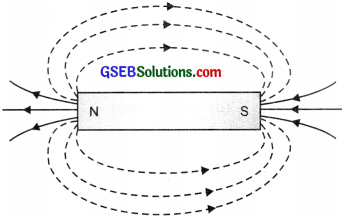Question 3.
List the properties of magnetic lines of force.
Properties of magnetic field lines of force:

•  Magnetic field lines travel from N-pole of the magnet towards S-pole, outside the magnet and from S-pole to N-pole inside the magnet.
• The strength of a magnet/magnetic field is given by how close the field lines are if the field lines are closer magnet is stronger.
• No two magnetic field lines ever intersect each other.

Question 4.
Why don’t two magnetic lines of force intersect each other?
The magnetic field lines never intersect each other as an intersection of the field lines means the magnetic field at that point has two directions, which is not possible because the resultant force on a pole (north/south) at any point can only be in one direction.Question 5.
Consider a circular loop of wire lying in the plane of the table. Let the current pass through the loop clockwise. Apply the right-hand nile to find out the direction of the magnetic field inside and outside the loop.Question 6.
The magnetic field in a given region is uniform. Draw a diagram to represent it.The magnetic field in a given region is uniform can be represented by showing parallel lines at equidistance.

Question 7.
Choose the correct option.
The magnetic field inside a long straight solenoid-carrying current
(a) is zero.
(b) decreases as we move towards its end.
(c) increases as we move towards its end.
(d) is the same at all points.
(d) is the same at all points.

Question 8.
Which of the following property of a proton can change while it moves freely in a magnetic field? (There may be more than one correct answer).
(a) mass
(b) speed
(c) velocity
(d) momentum
Velocity and momentumQuestion 9.
In activity 13.7 how do we think the displacement of rod AB will be affected if

1. current in rod AB is increased
2. a stronger horse-shoe magnet is used and
3. length of the rod AB is increased.

1. If the current in rod AB is increased its displacement will increase.
2. If a stronger horse-shoe magnet is used then the displacement of rod AB will increase.
3. If the length of the rod AB is an increased force acting on it will increase and the displacement of the rod also increases.

Question 10.
A positively charged particle (alpha-particle) projected towards the west is deflected towards the north by a magnetic field. The direction of the magnetic field is –
(a) towards south
(b) towards east Magnetic field
(c) downward
(d) upward
(c) downward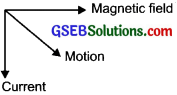Question 11.
State Flemings left-hand rule. (CBSE 2015)
According to this rule, stretch the thumb, forefinger and middle finger of left hand such that they are mutually perpendicular. If the first finger points in the direction of the magnetic field, the middle points in the direction of flow of current, then the thumb points in the direction of motion i.e., the force acting on the conductor.Question 12.
What is the principle of an electric motor?
The principle of an electric motor is based on Fleming’s left-hand rule. i.e., a current-carrying conductor experiences a force when placed in a magnetic field.

Question 13.
What is the role of the split ring in an electric motor?
In an electric motor, the split ring acts as a commutator. A split ring is connected to the ends of the coil and the direction of current flowing in motor coil reverses after every half turn. Which results in the continuous rotation of the coil and the ends.

Question 14.
Explain different ways to induce a current in a coil.
We can induce a current in a coil –

• by moving a coil in a magnetic field or by changing the magnetic field around it.
• by increasing the number of coils.

Question 15.
State the principle of an electric generator.
In an electric generator, mechanical energy is used to rotate a conductor in a magnetic field to produce electricity. Fleming’s right-hand rule is used where motion and the magnetic field is given and the current is induced.Question 16.
Name some sources of direct current.
Dynamo and battery cells.

Question 17.
Which sources produce alternating current?
Electric Generator with split rings

Question 18.
Choose the correct option.
A rectangular coil of copper wire is rotated in a magnetic field. The direction of the induced current changes once in each
(a) two revolutions
(b) one revolution
(c) half revolution
(d) one-fourth revolution
(c) half revolution

Question 19.
Name two safety measures commonly used in electric circuits and appliances.

• Use earthing wiring for metallic body electrical appliances.
• Use miniature circuit breakers, (MCB) i.e., fuse

Question 20.
An electric oven of 2 kW power rating is operated in a domestic electric circuit (220 V) that has a current rating of 5 A. What result do you expect? Exp1ain.
P = 2kW = 2000 W
V = 220V
I = 5A

as, P = Vl
2000 W = 220 x 5
2000 W = 1100Here power and value of VI are not the same that means the current required for the oven is more than 5 A that the circuit can supply.

Question 21.
What precautions should be taken to avoid the overloading of domestic electric circuits?

• Two separate circuits should be used one of 5 A current and other 15 A.
• For both 5 A and 15 A circuits, the fuse should be installed.
• Parallel circuits should be used.
• Never use too many electrical appliances at one point.

In-Text Activities Solved

Activity 13.1

• Take a straight thick copper wire and place it between the points X and Y in an electric circuit as shown on the next page. The wire XY is kept perpendicular to the plane of the paper.
• Magnetic Effects of Electric Current 141 Horizontally place a small compass near to this copper wire. See the position of its needle.
• Pass the current through the circuit by inserting the key into the plug.
• Observe the change in the position of the compass needle.

Observation: As shown in the figure – the current flows from +ve to -ve and the current flows from X to Y. The needle deflects to right.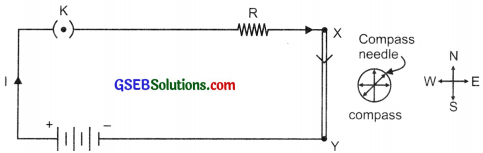Compass needle deflects as current passes through a metallic conductor. It means that the electric current through the wire has produced a magnetic effect.Activity 13.2

• Fix a sheet of white paper on a drawing board using some adhesive material.
• Place a bar magnet in the centre of it.
• Sprinkle some iron filings uniformly around the bar magnet. A salt-sprinkler may be used for this purpose.
• Now tap the board gently.

Observation:

• The iron filings arrange themselves in a pattern as shown in the figure.
• The lines along with which the iron filings align themselves represent magnetic field lines.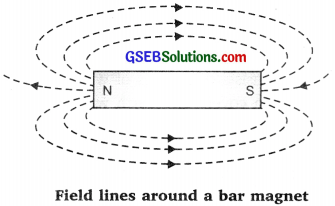Activity 13.3

• Take a small compass and a bar magnet.
• Place the magnet on a sheet of white paper fixed on a drawing board, using some adhesive material.
• Mark the boundary of the magnet.
• Place the compass near the north pole of the magnet. The south pole of the needle
• points towards the north pole of the magnet. The north pole of the compass is directed away from the north pole of the magnet.
• Mark the position of two ends of the needle.
• Now move the needle to a new position such that its south pole occupies the position previously occupied by its north pole.
• In this way, proceed step by step till you reach the south pole of the magnet.
• Join the points marked on the paper by a smooth curve. This curve represents a field line.
• Repeat the above procedure and draw as many lines as you can. A pattern will be formed as shown in the figure.
• These lines represent the magnetic field around the magnet. These are known as magnetic field lines.
• Observe the deflection in the compass needle as you move it along the field line. The deflection increases as the needle is moved towards the poles.Deflection of compass needle used to draw field lines around a bar magnet.Activity 13.4

• Take a long straight copper wire, two or three cells of 1.5 V each, and a plug key.
• Connect all of them in scenes, as shown in the figure on the next page.
• Place the straight wire parallel to and over a compass needle.
• Plug the key in the circuit.
• Observe the direction of the deflection of the north pole of the needle. If the current flows from north to south, as shown in the figure on the next page, the north pole of the compass needle would move towards the east.
• Replace the cell connections in the circuit. This would result in the change of the direction of current through the copper wire, that is, from south to north.
• Observe the change in the direction of deflection of the needle. You will see that now the needle moves in the opposite direction, that is, towards the west. It means that the direction of the magnetic field produced by the electric current is also reversed.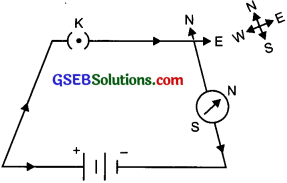Current flows from North to South. Needle deflects towards E(east) NSECurrent flows from South to North. Needle deflects towards W(west)

Activity 13.5

• Take a battery of I a variable resistance or a rheostat, an ammeter (0 -5 A), a plug key and a long straight thick copper wire.
• Insert the thick wire through the centre, normal to the plane of rectangular cardboard. Take care that the cardboard is fixed and does not slide up or down.
• Connect the copper wire vertically between the points X and Y as shown in the figure, in series with the battery, plug and key.
• Sprinkle some iron filings uniformly on the cardboard.
• Keep the variable of the rheostat at a fixed position and note the current through the ammeter.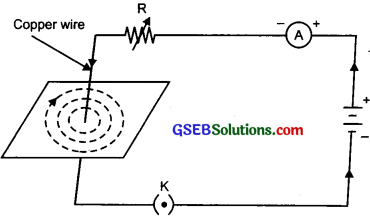A pattern of concentric circles Indicating the field lines of a magnetic field around a straight conducting wire. The arrow shows the direction of the field lines.
• Close the key so that a current flows through the wire. Ensure that the copper wire placed between the points X and Y remains vertically straight.
• Gently tap the cardboard a few times. Observe the pattern of the iron filings. The iron filings align themselves showing a pattern of concentric circles around the copper wire.
• These concentric circles represent the magnetic field lines.
• With the help of a compass needle, the direction of the field lines can be observed.
• When the current through the wire has reversed the direction of magnetic field lines also gets reversed.

Activity 13.6

• Take rectangular cardboard having two holes. Insert a circular coil having a large number of turns through them, normal to the plane of the cardboard.
• Connect the ends of the coil in series with a battery a key and a rheostat.
• Sprinkle iron filings uniformly on the cardboard. Plug the key.
• Tap the cardboard gently a few times. Note the pattern of the iron filings.

Observation: The magnetic field produced is much stronger and the iron filings show the pattern as shown in the figure below.The magnetic field produced by a current-carrying circular coil.Activity 13.7

• Take a small aluminium rod AB. Using two connecting wires suspend it horizontally from a stand, as shown in the figure.
• Place a strong horseshoe magnet in such a way that the rod lies between the two poles with the magnetic field directed upwards. For this put the north pole of the magnet vertically below and south pole vertically above the aluminium rod.
• Connect the aluminium rod in series with a batter3 a key and a rheostat.
• Now pass the current through the aluminium rod from end B to end A.
Observation: It is observed that the rod is displaced towards the left. The rod gets displaced.
• Reverse the direction of current flowing through the rod and observe the direction of its displacement. It is now towards the right.
• The rod gets displaced due to the force exerted on the current-carrying aluminium rod when it is placed in a magnetic field.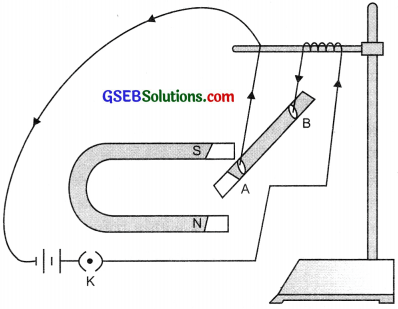current-carrying rod AB, experiences a force perpendicular to its length and the magnetic field.

Activity 13.8

• Take a coil of wire AB having a large number of turns. Connect the ends of the coil to a galvanometer as shown in the fig.
• Take a strong bar magnet and move its north pole towards the end B of the coil.
• Observation: Galvanometer shows the deflection.
• This indicates the presence of a current in the coil AB. The deflection becomes zero the moment the motion of the magnet stops.
• Now withdraw the north pole of the magnet away from, the coil. The galvanometer deflects towards the left, showing that the current is now set up in the direction opposite to the first.
• Place the magnet stationary at a point near to the coil, keeping its north pole towards the end B of the coil. We see that the galvanometer needle deflects towards the right when the coil is moved towards the north pole of the magnet. Similarly, the needle moves towards the left when the coil is moved away.
• When the coil is kept stationary with respect to the magnet, the deflection of the galvanometer drops to zero.

Conclusion: Motion of magnet with respect to the coil produces an induced potential difference, which sets up an induced electric current in the circuit.Moving a magnet towards a coil sets up a current In the coil circuit, as Indicated by deflection in the galvanometer needle.

Activity 13.9

• Take two different coils of copper wire having a large number of turns (say 50 and 100 turns respectively). Insert them over a non-conducting cylindrical roll, as shown in the figure.
• Connect the coil-i, having a larger number of turns, in series with a battery and a plug key. Also, connect the other coil-2 with a galvanometer as shown in the figure.
• Plugin the key. Observe the galvanometer. The needle of the galvanometer instantly jumps to one side and just as quickly returns to zero, indicating a momentary current in coil-2.
• Disconnect coil-i from the battery. The needle momentarily moves but to the opposite side. It means that now the current flows in the opposite direction in coil-2.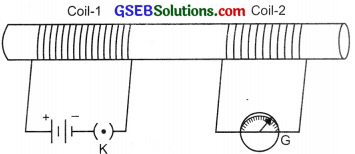Current Is induced in coil-2 when current in coil-I is changed.

Observation: We observe that as soon as the current in coil-i reaches either a steady value or zero, the galvanometer in coil-2 shows no deflection.
Conclusion: A potential difference is induced in the coil-2 whenever the electric current through the coil-1 is changing. Coil-1 is called the primary coil and coil-2 is called the secondary coil.

### Gujarat Board Class 10 Science Magnetic Effects of Electric Current Textbook Questions and Answers

Question 1.
Which of the following correctly describes the magnetic field near a long straight wire?
(a) The field consists of straight lines perpendicular to the wire.
(b) The field consists of straight lines parallel to the wire.
(c) The field consists of radial lines originating from the wire.
(d) The field consists of concentric circles centred on the wire.
(d) The field consists of concentric circles centred on the wire.

Question 2.
The phenomenon of electromagnetic induction is
(a) the process of charging a body.
(b) the process of generating a magnetic field due to current passing through a coil.
(c) producing induced current in a coil due to relative motion between a magnet and the coil.
(d) the process of rotating a coil of an electric motor.
(c) producing induced current in a coil due to relative motion between a magnet and the coil.Question 3.
The device used for producing electric current is called a
(a) generator
(b) galvanometer.
(c) ammeter
(d) motor.
(a) generator

Question 4.
The essential difference between an AC generator and a DC generator is that
(a) AC generator has an electromagnet while a DC generator has a permanent magnet.
(b) DC generator will generate a higher voltage.
(c) AC generator will generate a higher voltage.
(d) AC generator has split rings while the DC generator has a commutator.
(d) AC generator has split rings while the DC generator has a commutator.

Question 5.
At the time of short circuit the current in the circuit
(a) reduces substantially
(b) does not change.
(c) increases heavily
(d) vary continuously.
(c) increases heavily.

Question 6.
State whether the following statements are true or false.
(a) An electric motor converts mechanical energy into electrical energy
(b) An electric generator works on the principle of electromagnetic induction.
(c) The field at the centre of a long circular coil carrying current will be parallel straight lines.
(d) A wire with green insulation is usually the live wire of electric supply.
(a) False
(b) True
(c) True
(d) False.Question 7.
List two methods of producing magnetic fields.
The magnetic field can be produced by any of the following methods:

• Any magnet – bar magnet, horseshoe magnet or round magnet can be used.
• A wire carrying current produces a field around it.
• A loop or solenoid carrying current.

Question 8.
How does a solenoid behave like a magnet? Can you determine the north and south poles of a current-carrying solenoid with the help of a bar magnet? Explain.
A coil of many circular turns of insulated copper wire wrapped closely in the shape of a cylinder is called a solenoid. One end of the solenoid behaves as a magnetic north pole, while the other end behaves as the south pole. The field lines inside the solenoid are in the form of parallel straight lines.

By taking a bar-magnet with known north poles near one end of the solenoid and if it shows repulsion then that end of solenoid is the north pole and the other end is the south pole. The property of magnet le. like poles repel and unlike poles attract is used for the determination of poles of the solenoid.Question 9.
When is the force experienced by a current-carrying conductor placed in a magnetic field largest?
According to Flemings left-hand rule the force experienced by a current-carrying conductor placed in a magnetic field is largest when they both are perpendicular to each other.

Question 10.
Imagine that you are sitting in a chamber with your back to one wall. An electron beam, moving horizontally from the back wall towards the front wall is deflected by a strong magnetic field to your right side. What is the direction of the magnetic field?
According to Fleming’s left-hand rule, the direction of the magnetic field is downwards.Question 11.
Draw a labelled diagram of an electric motor. Explain its principle and working. What is the function of a split ring in an electric motor?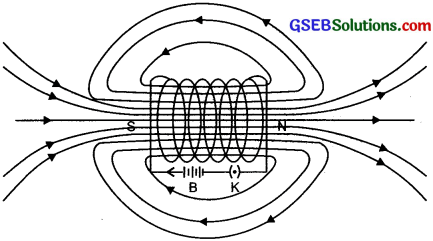Principle: A current-carrying conductor when placed at a right angle to a magnetic field, experiences a force due to a which we get motion. The direction of the force is given by Fleming’s left-hand rule.

Working: Current in the coil ABCD enters from the source battery through conducting brush X and flows back to the battery through brush Y. The current flows from arm A to Barm and then C to D, the direction of flow of current in both arms is opposite.

As per Fleming’s left-hand rule, the force acting on arm AB pushes it downwards while the force acting on CD pushes it upwards. Thus, the coil and the axle rotate anti-clockwise. Due to the action of split-ring commutator at half rotation, split rings P and Q change their contacts with brushes. Now P makes contact with Y and Q with X.

As a result, current begins to flow in coil along DCBA, As a result now arm AB is being pushed upward and arm CD downward by the magnetic force. So coil rotates half a turn more in the same direction. This reversing of current direction is repeated at each half rotation and so the coil continues to rotate in the same direction. The split ring helps in changing the direction of the current.

Question 12.
Name some devices in which electric motors are used.
The electric motor is used in all such devices where electric energy is used, converted into mechanical energy to get the motion of the machine. E.g. it is used in electric fans, mixer grinders, coolers, A.C., washing machines, computers etc.

Question 13.
A coil of insulated copper wire is connected to a galvanometer. What will happen if a bar magnet is –

1. pushed into the coil
2. withdrawn from inside the coil
3. held stationary inside the coil?

1.  If a bar magnet is pushed into the coil of insulated copper wire, the galvanometer shows the deflection as current is induced in the coil.
2. When the bar magnet is withdrawn from the coil the galvanometer shows deflection again but now to the opposite side.
3. When the bar magnet is held stationary inside the coil, the galvanometer does not show any deflection no induced current.

Question 14.
Two circular coils A and B are placed close to each other. If the current in the coil A is changed, will some current be induced in coil B? Give reason.
The current if changed in coil A. Some current will be induced in coil B.
Reason:  When the current in coil A is changed, the magnetic field around it also changes. As the coil B is placed very close to the coil A, the magnetic field lines around the B coil also changes due to this as the current is induced in it.

Question 15.
State the rule to determine the direction of a

1. magnetic field produced around a straight conductor carrying current.
2. the force experienced by a current-carrying straight conductor placed in a magnetic field which is perpendicular to it, and
3. current induced in a coil due to its rotation in a magnetic field.

1. To know the direction of the magnetic field produced around a straight conductor- carrying current. The rule used is – Right-hand thumb rule’.
2. Fleming’s left-hand rule is used to find the direction of force experienced by a current-carrying straight conductor when placed in a magnetic field, which is perpendicular to it.
3. Fleming’s right-hand rule is used to determine the direction of current induced in a coil due to its rotation in a magnetic field.

Question 16.
Explain the underlying principle and working of an electric generator by drawing a labelled diagram. What is the function of the brushes?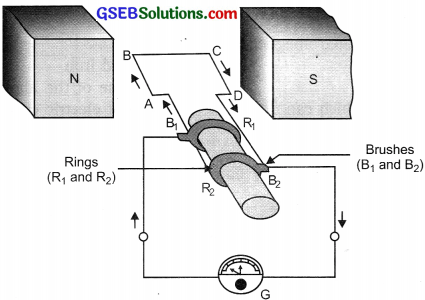Principle: An electric generator works on the principle of electromagnetic induction. When a coil is rotated between the magnet or when the magnet is rotated in and out of the coil the current is induced in the coil and the direction of curreñt is given by Fleming’s right-hand rule.

Working: As shown in the figure, when the axle attached to the two rings is rotated such that arm AB moves up and arm CD moves down in the magnetic field produced by the permanent magnet i.e. ABCD rotates clockwise. By Fleming’s right-hand rule, the induced currents are set up in these arms and flow in the direction ABCD, which flows from B2 to B1.After half a rotation, arm CD starts moving up and AB moves down. As the directions of the induced currents in both the arms change current is induced in direction DCBA, which further flows from B1 to B2 externally. After every half rotation the polarity of the current in arms changes. Such current which changes direction after equal intervals of time is called an alternating current (AC).

Brushes: are used to transmit current-induced externally from coil ABCD to the external circuit.

Question 17.
When does an electric short circuit occur?
When the insulation of wire gets damaged and this naked wire comes in contact with other such wire i.e. live wire and neutral wire comes in contact, the current flowing in the circuit rises and short-circuiting occurs.

Question 18.
What is the function of an earth wire?
Why is it necessary to earth metallic appliances? (CBS E 2011)
The earth wire is connected as a safety measure with all electrical appliances that have metallic body e.g., microwave, electric press, toaster, geyser, cooler, AC, etc. The earth wire provides a low resistance conducting path for electric current. If there is any leakage of current then the user would not get any current because the current flows down into the earth and keeps the potential of the appliance and earth same.

### Gujarat Board Class 10 Science Magnetic Effects of Electric Current Additional Important Questions and Answers

Question 1.
What does an electric current-carrying wire behave like?
It behaves like a magnet.

Question 2.
Define magnetic field.
The region around a magnet in which the force of attraction or repulsion produced by the magnet can be detected is a magnetic field.

Question 3.
Define field lines.
Fieldline is a path along which a hypothetical free north pole would tend to move.

Question 4.
What kind of quantity is a magnetic field?
It is a vector quantity as it has both magnitude and direction.Question 5.
What is a compass needle?
A compass needle is a small bar magnet.

Question 6.
What are the properties of magnetic field lines?

• Magnetic field lines emerge from North pole and merge at the South Pole outsîde the magnet and inside the bar magnet from South pole to North pole.
• Magnetic field lines never intersect each other because at the point of intersection the compass needle would point in two directions which is not possible.
• The magnetic field is stronger at the poles than ¡n the middle. The direction of the magnetic field is indicated by the arrow in the line at any point (tangent).

Question 7.
On which factors do the magnetic field due to current carrying conductor depend?
The magnitude of the magnetic field produced at the given point:

• Increases as the current through wire increases.
• Decreases as the distance from the wire increases.

Question 8.
Name and state the rule used to determine the direction of the field in a current-carrying straight conductor.
The rule is – Right-hand thumb rule.

Question 9.
Draw the magnetic field lines due to current through a circular loop.
Magnetic lines of force are circular near the wire became parallel at the middle point of the coil.Question 10.
What is a solenoid?
The solenoid is a long coil of many turns of insulated copper wire wrapped in the shape of a cylinder.

Question 11.
How is solenoid different from a coil?
The solenoid is different from a circular coil in the sense that the length of the solenoid is much greater than its diameter.Question 12.
What is similar between solenoid and bar magnet?
The magnetic field produced by a solenoid is similar to the bar magnet.

Question 13.
What is an electromagnet?
A magnet formed temporarily due to the magnetic field of current is called an electromagnet.

Question 14.
Name and state rule used to determine the force on a current-carrying conductor in a magnetic field.
Force on a current-carrying conductor which is placed perpendicular to the direction of the magnetic field is given by Fleming’s Left Hand Rule. According to this rule, stretch the thumb, fore-finger and the middle finger of the left hand such that they are mutually perpendicular. 1f the fore-finger points in the direction of magnetic fields and middle finger in the direction of the current, then the thumb will point in the direction of motion or force acting on the conductor.Question 15.
On what factor does strength of the magnetic field of a solenoid depend?
Strength of the magnetic field of a solenoid is proportional to the number of turns of coil and magnitude of the current.

Question 16.
Name some devices that use current-carrying conductors and magnetic field.
Electric motor, electric generator, loudspeaker and measuring instruments.

Question 17.
How is magnetism helpful in medicine?
When we touch something our nerves carry an electric impulse to the muscles we need to use, this impulse produced is a temporary magnetic field. The magnetic field inside the body forms the basis of obtaining images of different body parts. This is done by using a technique called Magnetic Resonance Imaging (MRJ). Analysis of these images helps in medical diagnosis.

Question 18.
Name two organs in the human body where the magnetic field produced is significant.
Two organs are heart and brain.

Question 19.
What is an electric motor? Name some devices where an electric motor is used.
The electric motor is a device which converts electric energy into mechanical energy. The devices which use electric motor are an electric fan, mixer blender, water pumps, washing machine, refrigerator etc.

Question 20.
On what principle does electric motor work?
When the rectangular coil is placed in a magnetic field and the current passes through it, coil experiences a torque which rotates it continuously.Question 21.
With the help of a diagram explain the working of an electric motor.Principle: A current-carrying conductor when placed at a right angle to a magnetic field, experiences a force due to a which we get motion. The direction of the force is given by Fleming’s left-hand rule.

Working: Current in the coil ABCD enters from the source battery through conducting brush X and flows back to the battery through brush Y. The current flows from arm A to Barm and then C to D, the direction of flow of current in both arms is opposite.

As per Fleming’s left-hand rule, the force acting on arm AB pushes it downwards while the force acting on CD pushes it upwards. Thus, the coil and the axle rotate anti-clockwise. Due to the action of split-ring commutator at half rotation, split rings P and Q change their contacts with brushes. Now P makes contact with Y and Q with X.

As a result, current begins to flow in coil along DCBA, As a result now arm AB is being pushed upward and arm CD downward by the magnetic force. So coil rotates half a turn more in the same direction. This reversing of current direction is repeated at each half rotation and so the coil continues to rotate in the same direction. The split ring helps in changing the direction of the current.

Question 22.
What is the role of the split ring in an electric motor?
The role of a split ring is to reverse the direction of current flowing through the coil.

Question 23.
Define electromagnetic induction.
The phenomena of inducing an electric current in a coil by changing magnetic field around it is called electromagnetic induction.

Question 24.
Who discovered that mechanical motion can produce current?
Michael Faraday in 1831 discovered that mechanical motion can produce current.

Question 25.
What is a galvanometer?
A galvanometer is an instrument that can detect the presence of a current in a circuit.

Question 26.
Name and state the rule used to determine the direction of the induced current.
The direction of the induced current is given by Fleming’s right-hand rule according to this rule, stretch the thumb, fore-finger and the middle finger of the right hand such that they are mutually perpendicular. If the forefinger points in the direction of the magnetic field the thumb show the direction of motion and middle finger points in the direction of induced current.Question 27.
What is an electric generator?
It is a device that converts mechanical energy into electrical energy.

Question 28.
Draw a labelled diagram of a generator.
Refer Ans.16 in NCERT textual questions solved

Question 29.
What is the difference between AC generator and DC generator?
AC generator produces a current which changes its direction after equal intervals of time and DC generator produces a current which is unidirectional.

Question 30.
What is an electric fuse?
An electric fuse is a safety device made of thin wire of tin or lead-tin alloy having a low melting point which breaks the circuit when current exceeds safety limit.

Question 31.
Name the scientist who discovered the magnetic effects of current.
Hans Christian Oersted.

Question 31.
Name the scientist who discovered the magnetic effects of current.
Hans Christian Oersted .

Question 32.
Will current-carrying wire experience a force when kept parallel to the magnetic field?
No.

Question 33.
What kind of a motor is used in (a) a fan (b) a battery-operated toy?
(a) AC motor (b) DC motorQuestion 34.
If a current-carrying solenoid is suspended freely how will it come at rest?
In the north-south direction like a bar magnet.

Question 35.
Distinguish between permanent magnet and electromagnet.
Permanent magnet:

• Polarity cannot be changed
• Strength remains constant

Electromagnet:

• Polarity can be changed by reversing current flow.
• Strength can be changed by varying the magnitude of the current.

Question 36.
Distinguish between DC and AC.
DC:

• Unidirectional flow of current.
• Cells and batteries produce DC.

AC:

• Current changes its direction in a cycle.
• Dynamo produces AC.

Question 37.
What are the two most commonly used domestic circuit?

• 5 A for low power rating appliances.
• 15 A for high power rating appliances.

Question 38.
What is an earth wire?
An earth wire is connected to the metallic body of the appliances. This provides a low resistance conducting path for current and any leakage is safely conducted to earth reducing the severity of electric shock.Question 1.
State Fleming’s left-hand rule.
It states that hold the fore-finger, middle finger and thumb of the left hand mutually perpendicular to each other. If the fore-finger shows the direction of the magnetic field and the middle finger that of the current, then the thumb will point towards
the direction of motion of the conductor.

Question 2.
Why do we use the power supply of two different current ratings at our homes?
Each electrical appliance has its rating and draws current accordingly for its functioning. To avoid overloading and short-circuiting two separate circuits are used in the electrical wiring at home, one of 15 A for appliances with high power ratings and another of 5 A for bulbs, tubers, fans etc. electrical fuses of appropriate capacities are used ahead of each electric circuit as a safety measure.

Question 3.
Give four features of domestic electric wiring.

• The circuit is parallel to each other.
• Each circuit consists of an on-off switch.
• Two separate circuits are used, one of 15 A and another of 5 A.
• An electric fuse is connected to each circuit.

Question 4.
Give two differences between the electric motor and generator.
Electric motor:

• It works on the principle of Fleming’s left-hand rule.
• It uses the current and magnetic field to produce motion. –

Electric generator:

• k works on the principle of Fleming’s right-hand rule.
• k uses motion and magnetic field to induce a current.

Question 5.
In what ways can the magnitude of the induced current be increased?
The magnitude of the induced current can be increased by

• increasing the number of coils of the wire.
• by increasing the power of the magnet.Question 6.
Why do we prefer AC for transmission to long distances while most of the appliances are based on DC?
Transmission of current on long distant can result in a lot of loss of electrical energy as the energy gets transmitted into heat energy. Whereas in the case of AC current the loss of electrical energy is less as the wire gets less heated.

Question 7.
Give two serious hazards of electricity.

• Death due to shock.

Question 8.
(a) What would be the frequency of an alternating current if its direction changes after every 0.01 second?
(b) Which out of the two requires thin fuse wire – to draw 5 A or 15 A of current?
(a) Time required to complete one cycle = 0.01 x 2 = 0.02s
So, cycles completed in is = 1/0.02 = 50 Hz.
Frequency would be 50 Hz.
(b) Thin fuse wire will be used in 5 A circuit.Question 9.
Draw a schematic diagram of domestic wiring system and write its main features.Main features:

• Connect circuits in parallel.
• Each circuit has MCB (miniature circuit breaker) act as a small individual fuse.
• Two separate circuits of 5 A and 15 A are used.
• Earth wire is connected to the metallic body appliances.

Question 10.
Name the components of electric motor that is used for the application of Fleming’s left-hand rule.
For electric motor we use –

• A coil having a large number of turns, the magnetic force due to all wires is added up to get the very strong force.
• Electromagnet: By increasing the current strength of an electromagnet can be increased and the motion can be increased.
• A soft iron in an electric motor will help in increasing the power of the motor.

Question 11.
Give three important features of the magnetic field due to current carrying solenoid coil.
Important features of the magnetic field due to a current-carrying solenoid coil are:

• The magnetic field lines inside the solenoid are nearly straight and parallel.
• Solenoid behaves like a bar magnet as the magnetic field lines are strong at the poles.
• The solenoid also shows the directive and attractive properties of a magnet.

Question 12.
Draw magnetic field through and around a solenoid carrying electric current.Coiled loop of wire is – solenoid
B – battery     South and North pole – S and NQuestion 13.
Name the factors on which force produced due to magnetic field depends.
The factors on which force produced due to magnetic field depends are:

• length of the conductor.
• the amount of current flowing.
• the strength of the magnetic field.
• the direction of the current and magnetic field, if are at right angles then the maximum amount of force is produced.

Question 14.
(a) Distinguish between the terms overloading and short-circuiting as used in domestic circuits.
(b) Why are the coils of electric toasters made of an alloy rather than a pure metal?
(a) Overloading is the act of connecting many devices to a single supply point and trying to draw a large amount of current. Short-circuiting is a situation of the flow of large current due to malfunctioning of devices or the joining of the life and the neutral wire

(b) Alloys have higher resistivity and do not oxidise or burn readily at high temperatures.

Question 15.
When is the force experienced by a current-carrying conductor placed in a magnetic field the largest?
When the length (current direction) and magnetic field B are in perpendicular
direction, the force experienced is maximum and is given by F = lB.Question 16.
(a) What is the standard colour code followed for
1. Live
2. Neutral
3. Earth
wires used in electric circuits?
(b) Which part of an electric appliance is earthed and why?
1. The standard colour code for live wire is red
2. neutral wire is blue and the
3. earth wire is green.

(b) The electrical appliances with the metallic body have to earth and it is the metallic plate that is earthed through the plug point.Question 17.
In a power plug why is the one point longer as shown in the figure than the other two?
The power plug is generally used for connecting the appliances with the metallic body and the longest point on the top is used for earthing. This is a safety measure so that when you plug such electrical appliances the first connection should be of earthing which will prevent a person from shocks.Question 18.
Name the materials used to make an electromagnet. Explain how you can make one in the lab.
To make an electromagnet one can use steel or iron as a core. The making of the electromagnet in the lab needs following material, the source for current, battery, metals wire with no insulation and hand gloves. Make a loop. Like a solenoid, wrap the soft steel core or iron and connect its terminals to the terminals of the battery. Allow the current to pass through it and the core inside now behaves like a magnet and can attract the magnetic materials.

Question 19.
Name the magnetic materials.
The magnetic materials are iron, nickel and cobalt.

Question 20.
Suggest two ways of increasing the strength of the magnetic field lines produced by a circular coil carrying current.
The two methods to increase the strength of the magnetic field lines of the coil is:
(a) Increase the amount of current flowing through the coil.
(b) Increase the number of coils or turns in the wire.

Question 21.
State the difference in the electromagnet and permanent magnet
Electromagnet:

• It is a temporary magnet
• Can be demagnetized easily
• The strength of the magnet can be changed
• Its polarity can be changed and is made from soft iron

Permanent magnet:

• It is a permanent magnet
• Cannot be demagnetized easily
• The strength is fixed and cannot be changed
• Its polarity is fixed and is made using steel or Alnico.

Question 1.
(a) What is meant by a magnetic field?
(b) How is the direction of the magnetic field at a point determined?
(c) Describe an activity to demonstrate the direction of the magnetic field generated around a current-carrying conductor.
(d) What is the direction of the magnetic field at the centre of a current-carrying circular loop?
(a) The magnet exerts its influence in the region surrounding it the force of magnet can be detected in this region and is said magnetic field.
(b) The direction of the magnetic field at a point is determined by the compass needle.
(c) Activity 13.5 on page 511.
(d) It is in a straight line.Question 2.
(a) What is an electromagnet? What does it consist of?
(b) Name one material in each case which is used to make a
(i) Permanent magnet
(ii) Temporary magnet
(c) Describe an activity to show how can you make an electromagnet in your school laboratory.
(a) A magnet formed due to the magnetic field of electric current is called an electromagnet. It is a temporary magnet. It is made of winding wire over a core made of soft magnetic material. Once the current is switched off, electromagnet loses its magnetism.
(b) (i) Permanent magnet-alloys of aluminium, nickel and cobalt – alnico
(ii) Temporary magnet-soft iron
(c) To make an electromagnet in the laboratory: Take solenoid, soft iron, battery, key.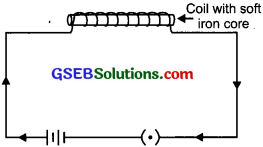Arrange the set up as shown in the figure. Allow the current to flow through the solenoid. Each turn of the solenoid produces a magnetic field. The magnetic field inside the solenoid is uniform hence the soft iron placed inside the solenoid gets magnetised, but once the current is switched off, it loses its magnetic power.

Question 3.
Describe an activity to show that a magnetic field is produced by an electric current
flowing through a circular coil of wire.
Aim: To show that the magnetic field is produced due to a circular coil carrying current.
Apparatus Required: Iron filings, a circular coil of known numbers of turns, cardboard, a stand, a key, a battery etc.Procedure:

• Attach the circular coil with a pair of holes in cardboard fitted in a stand.
• Connect the free ends of the circular coil to the battery through a key and a rheostat.
• Orient the coil exactly in the vertical plane such that the cardboard is in the horizontal plane.
• Spread uniformly iron filing on the cardboard.
• Plugin the key and observe the orientation of iron filing after slight tapping.
• Place a needle and indicate the direction of the magnetic field.
• Reverse the current and observe the needle.

Observations:
The orientation of iron filing observed on the cardboard are indicators of magnetic field lines.
They are observed to be:

• Circular near the wire.
• Straight at the centre.
• The field is perpendicular to the plane of the coil.
• For larger areas, the field at the centre is uniform.
• Field lines will enter into one plane and leave the other. (Enter on the side with the clockwise current).

Conclusion:
Presence of circular array and some straight array of iron filings at the middle confirms the presence of the magnetic field.